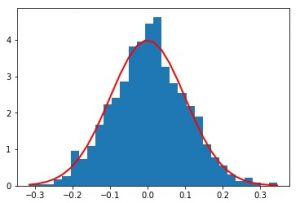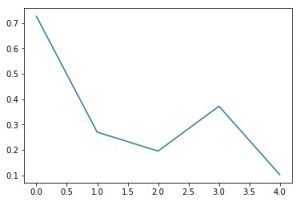Related Articles

# rand vs normal in Numpy.random in Python

• Last Updated : 17 Nov, 2020

In this article, we will look into the principal difference between the Numpy.random.rand() method and the Numpy.random.normal() method in detail.

• About random: For random we are taking .rand()
numpy.random.rand(d0, d1, …, dn) :
creates an array of specified shape and
fills it with random values.
Parameters :
```d0, d1, ..., dn : [int, optional]
Dimension of the returned array we require,

If no argument is given a single Python float
is returned.
```

Return :

```Array of defined shape, filled with random values.
```
• About normal: For random we are taking .normal()
numpy.random.normal(loc = 0.0, scale = 1.0, size = None) : creates an array of specified shape and fills it with random values which is actually a part of Normal(Gaussian)Distribution. This is Distribution is also known as Bell Curve because of its characteristics shape.
Parameters :
```loc   : [float or array_like]Mean of
the distribution.
scale : [float or array_like]Standard
Derivation of the distribution.
size  : [int or int tuples].
Output shape given as (m, n, k) then
m*n*k samples are drawn. If size is
None(by default), then a single value
is returned.
```

Return :

```Array of defined shape, filled with
random values following normal
distribution.
```

Code 1 : Randomly constructing 1D array

 `# Python Program illustrating``# numpy.random.rand() method``  ` `import` `numpy as geek``  ` `# 1D Array``array ``=` `geek.random.rand(``5``)``print``(``"1D Array filled with random values : \n"``, array)`

Output :

```1D Array filled with random values :
[ 0.84503968  0.61570994  0.7619945   0.34994803  0.40113761]

```

Code 2 : Randomly constructing 1D array following Gaussian Distribution

 `# Python Program illustrating``# numpy.random.normal() method``  ` `import` `numpy as geek``  ` `# 1D Array``array ``=` `geek.random.normal(``0.0``, ``1.0``, ``5``)``print``(``"1D Array filled with random values "``      ``"as per gaussian distribution : \n"``, array)``# 3D array``array ``=` `geek.random.normal(``0.0``, ``1.0``, (``2``, ``1``, ``2``))``print``(``"\n\n3D Array filled with random values "``      ``"as per gaussian distribution : \n"``, array)`

Output :

```1D Array filled with random values as per gaussian distribution :
[-0.99013172 -1.52521808  0.37955684  0.57859283  1.34336863]

3D Array filled with random values as per gaussian distribution :
[[[-0.0320374   2.14977849]]

[[ 0.3789585   0.17692125]]]
```

Code3 : Python Program illustrating graphical representation of random vs normal in NumPy

 `# Python Program illustrating``# graphical representation of ``# numpy.random.normal() method``# numpy.random.rand() method``  ` `import` `numpy as geek``import` `matplotlib.pyplot as plot``  ` `# 1D Array as per Gaussian Distribution``mean ``=` `0` `std ``=` `0.1``array ``=` `geek.random.normal(``0``, ``0.1``, ``1000``)``print``(``"1D Array filled with random values "``      ``"as per gaussian distribution : \n"``, array);`` ` `# Source Code : ``# https://docs.scipy.org/doc/numpy-1.13.0/reference/``# generated/numpy-random-normal-1.py``count, bins, ignored ``=` `plot.hist(array, ``30``, normed``=``True``)``plot.plot(bins, ``1``/``(std ``*` `geek.sqrt(``2` `*` `geek.pi)) ``*``          ``geek.exp( ``-` `(bins ``-` `mean)``*``*``2` `/` `(``2` `*` `std``*``*``2``) ),``          ``linewidth``=``2``, color``=``'r'``)``plot.show()`` ` ` ` `# 1D Array constructed Randomly``random_array ``=` `geek.random.rand(``5``)``print``(``"1D Array filled with random values : \n"``, random_array)`` ` `plot.plot(random_array)``plot.show()`

Output :

```1D Array filled with random values as per gaussian distribution :
[ 0.12413355  0.01868444  0.08841698 ..., -0.01523021 -0.14621625
-0.09157214]1D Array filled with random values :
[ 0.72654409  0.26955422  0.19500427  0.37178803  0.10196284]```

Important :
In code 3, plot 1 clearly shows Gaussian Distribution as it is being created from the values generated through random.normal() method thus following Gaussian Distribution.
plot 2 doesn’t follow any distribution as it is being created from random values generated by random.rand() method.

Note :
Code 3 won’t run on online-ID. Please run them on your systems to explore the working.
.
This article is contributed by Mohit Gupta_OMG 😀. If you like GeeksforGeeks and would like to contribute, you can also write an article using contribute.geeksforgeeks.org or mail your article to contribute@geeksforgeeks.org. See your article appearing on the GeeksforGeeks main page and help other Geeks.